# basic biomechanics levers.pptx

30 de Jan de 2023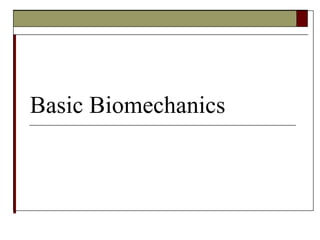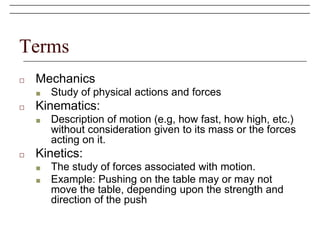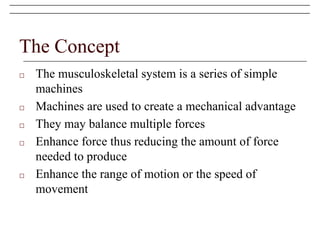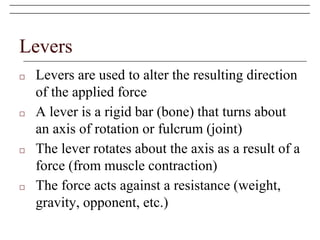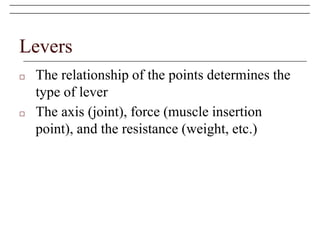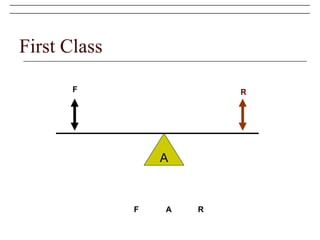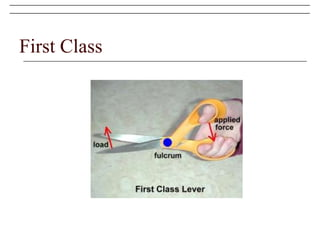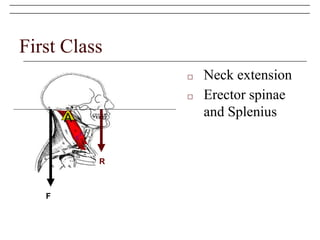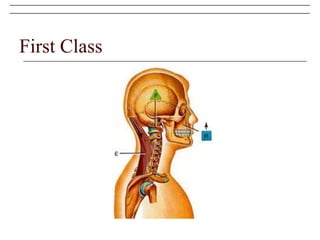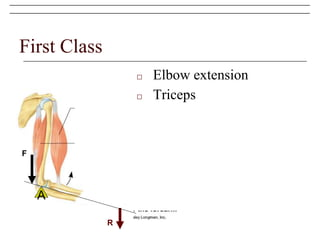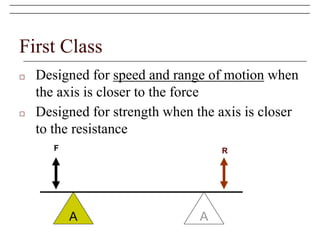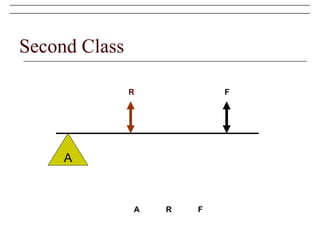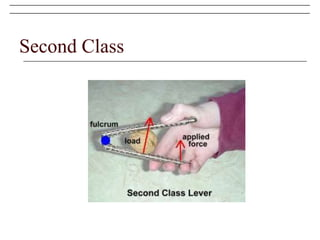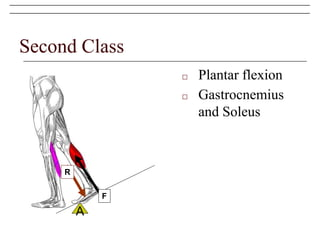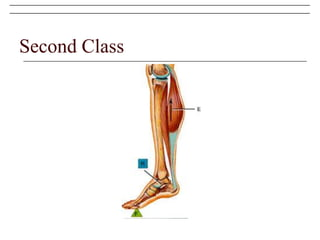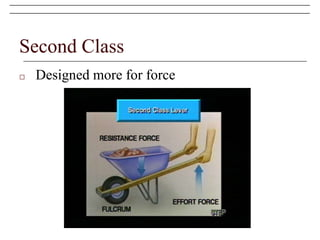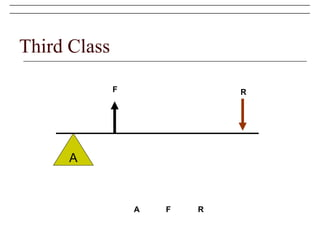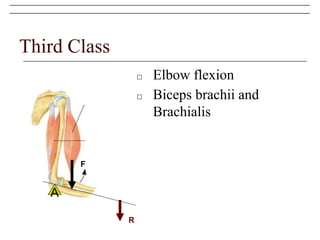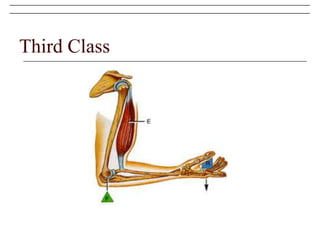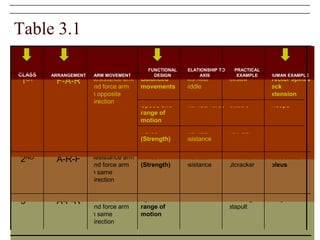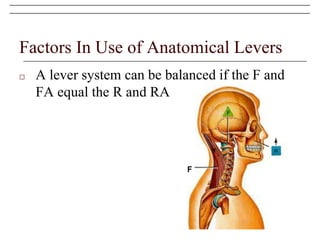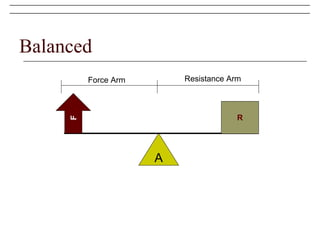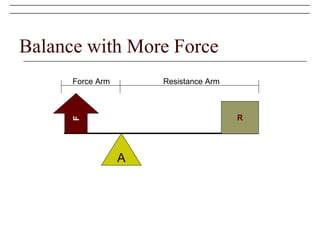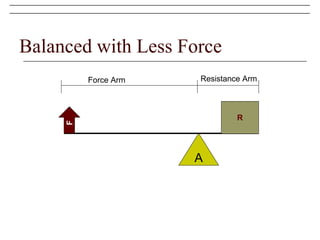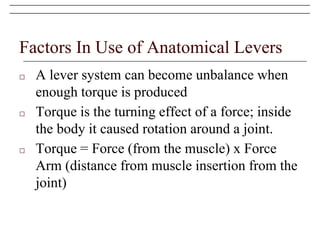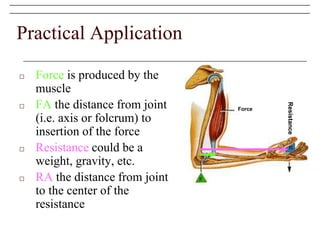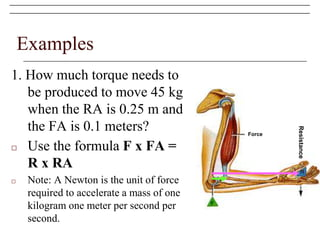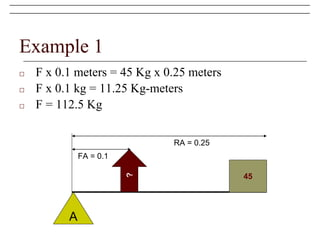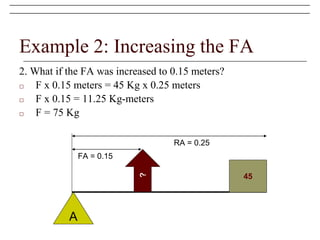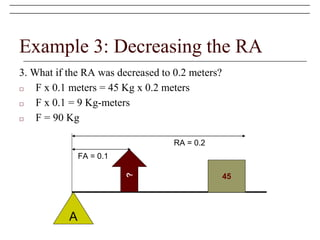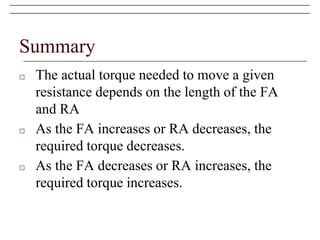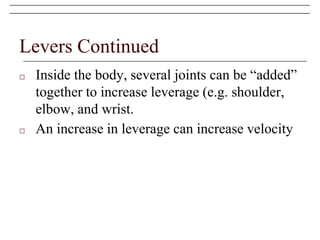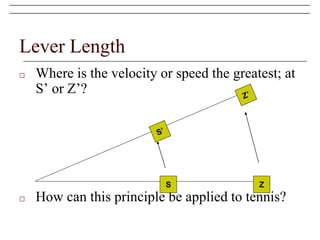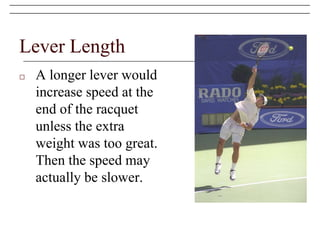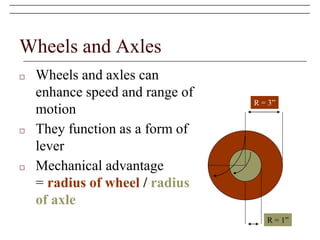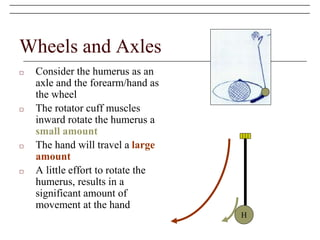1 de 38

### basic biomechanics levers.pptx

• 1. Basic Biomechanics
• 2. Terms □ Mechanics ■ Study of physical actions and forces □ Kinematics: ■ Description of motion (e.g, how fast, how high, etc.) without consideration given to its mass or the forces acting on it. □ Kinetics: ■ The study of forces associated with motion. ■ Example: Pushing on the table may or may not move the table, depending upon the strength and direction of the push
• 3. The Concept □ The musculoskeletal system is a series of simple machines □ Machines are used to create a mechanical advantage □ They may balance multiple forces □ Enhance force thus reducing the amount of force needed to produce □ Enhance the range of motion or the speed of movement
• 4. Levers □ Levers are used to alter the resulting direction of the applied force □ A lever is a rigid bar (bone) that turns about an axis of rotation or fulcrum (joint) □ The lever rotates about the axis as a result of a force (from muscle contraction) □ The force acts against a resistance (weight, gravity, opponent, etc.)
• 5. Levers □ The relationship of the points determines the type of lever □ The axis (joint), force (muscle insertion point), and the resistance (weight, etc.)
• 6. First Class A F R F A R
• 7. First Class
• 8. First Class □ Neck extension □ Erector spinae and Splenius A F R
• 9. First Class
• 10. First Class □ Elbow extension □ Triceps A F R
• 11. First Class □ Designed for speed and range of motion when the axis is closer to the force □ Designed for strength when the axis is closer to the resistance A F R A
• 12. Second Class A F R A R F
• 13. Second Class
• 14. Second Class □ Plantar flexion □ Gastrocnemius and Soleus A F R
• 15. Second Class
• 16. Second Class □ Designed more for force
• 17. Third Class A F R A F R
• 18. Third Class
• 19. Third Class □ Elbow flexion □ Biceps brachii and Brachialis A F R
• 20. Third Class
• 21. Table 3.1 Biceps brachii Shoveling dirt, catapult Axis near force Speed and range of motion Resistance arm and force arm in same direction A-F-R 3RD Gatroc and soleus Wheel barrow, nutcracker Axis near resistance Force (Strength) Resistance arm and force arm in same direction A-R-F 2ND Crow bar Axis near resistance Force (Strength) Triceps Scissors Axis near force Speed and range of motion Erector spinae neck extension Seesaw Axis near middle Balanced movements Resistance arm and force arm in opposite direction F-A-R 1ST HUMAN EXAMPLE PRACTICAL EXAMPLE RELATIONSHIP TO AXIS FUNCTIONAL DESIGN ARM MOVEMENT ARRANGEMENT CLASS
• 22. Factors In Use of Anatomical Levers □ A lever system can be balanced if the F and FA equal the R and RA F
• 23. Balanced A R F Force Arm Resistance Arm
• 24. Balance with More Force A R F Force Arm Resistance Arm
• 25. Balanced with Less Force A R F Force Arm Resistance Arm
• 26. Factors In Use of Anatomical Levers □ A lever system can become unbalance when enough torque is produced □ Torque is the turning effect of a force; inside the body it caused rotation around a joint. □ Torque = Force (from the muscle) x Force Arm (distance from muscle insertion from the joint)
• 27. Practical Application □ Force is produced by the muscle □ FA the distance from joint (i.e. axis or folcrum) to insertion of the force □ Resistance could be a weight, gravity, etc. □ RA the distance from joint to the center of the resistance Force Resistance
• 28. Examples 1. How much torque needs to be produced to move 45 kg when the RA is 0.25 m and the FA is 0.1 meters? □ Use the formula F x FA = R x RA □ Note: A Newton is the unit of force required to accelerate a mass of one kilogram one meter per second per second. Force Resistance
• 29. Example 1 □ F x 0.1 meters = 45 Kg x 0.25 meters □ F x 0.1 kg = 11.25 Kg-meters □ F = 112.5 Kg A 45 ? FA = 0.1 RA = 0.25
• 30. Example 2: Increasing the FA 2. What if the FA was increased to 0.15 meters? □ F x 0.15 meters = 45 Kg x 0.25 meters □ F x 0.15 = 11.25 Kg-meters □ F = 75 Kg A 45 ? FA = 0.15 RA = 0.25
• 31. Example 3: Decreasing the RA 3. What if the RA was decreased to 0.2 meters? □ F x 0.1 meters = 45 Kg x 0.2 meters □ F x 0.1 = 9 Kg-meters □ F = 90 Kg A 45 ? FA = 0.1 RA = 0.2
• 32. Summary □ The actual torque needed to move a given resistance depends on the length of the FA and RA □ As the FA increases or RA decreases, the required torque decreases. □ As the FA decreases or RA increases, the required torque increases.
• 33. Levers Continued □ Inside the body, several joints can be “added” together to increase leverage (e.g. shoulder, elbow, and wrist. □ An increase in leverage can increase velocity
• 34. Lever Length □ Where is the velocity or speed the greatest; at S’ or Z’? □ How can this principle be applied to tennis? S Z
• 35. Lever Length □ A longer lever would increase speed at the end of the racquet unless the extra weight was too great. Then the speed may actually be slower.
• 36. Wheels and Axles □ Wheels and axles can enhance speed and range of motion □ They function as a form of lever □ Mechanical advantage = radius of wheel / radius of axle R = 3” R = 1”
• 37. Wheels and Axles □ Consider the humerus as an axle and the forearm/hand as the wheel □ The rotator cuff muscles inward rotate the humerus a small amount □ The hand will travel a large amount □ A little effort to rotate the humerus, results in a significant amount of movement at the hand H